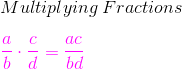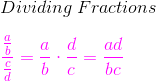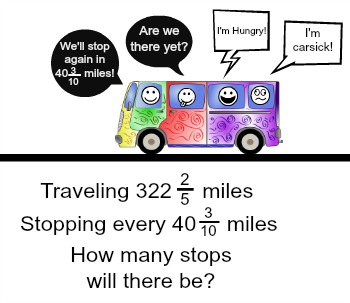# How to Divide Fractions with Unlike Denominators

Instructor: Laura Pennington

Laura received her Master's degree in Pure Mathematics from Michigan State University. She has 15 years of experience teaching collegiate mathematics at various institutions.

Once you know the underlying principle, diving with unlike denominators becomes easy. We'll learn how to divide fractions with unlike denominators, look at what's common between dividing fractions with like and unlike denominators, and see how they're applied in the world.

## Steps to Solve

We want to know how to divide fractions with unlike denominators. Some of you may be familiar with dividing fractions that have like denominators by simply canceling out the denominators and dividing the numerators.Well, as it turns out, this rule for dividing fractions with like denominators actually comes from the rule for dividing fractions in general. That is, there is a rule for dividing fractions regardless of if their denominators are the same or not!

To divide fractions, we need to now how to multiply fractions. Thankfully, multiplying fractions is probably the easiest operation to perform on fractions. To multiply fractions, we simply multiply their numerators together and multiply their denominators together, then simplify.Pretty simple, wouldn't you agree? Well, the great news is that to divide fractions, we turn the division problem into a multiplication problem. To do this, we simply multiply the numerator of the division problem by the reciprocal of the denominator of the division problem.

Wait! What's that fancy word there? What the heck is a reciprocal? Don't worry! The reciprocal of a fraction has a fancy name, but is actually quite simple - it just interchanges the numerator and denominator of a fraction. That is, the reciprocal of the fraction a/b is b/a. See? Simple, right?

Okay, now that we've got all of our parts, let's put them together to see how to divide fractions with unlike denominators! Like we said, to divide fractions, we simply multiply the numerator by the reciprocal of the denominator.We see that (a/b) / (c/d) = a/b * d/c = ad/bc. Now we can see where the rule for dividing fractions with like denominators comes from. Notice, if the denominators are the same, we have (a/b) / (c/b) = a/b * b/c = ab / bc. We see the b 's, or the denominators, cancel out to give a/c.

We see that dividing fractions with like or unlike denominators is actually the exact same process, and thankfully, the process is pretty easy!

## Solution

To divide fractions with unlike denominators, we turn the division problem into a multiplication problem by multiplying the numerator of the division problem by the reciprocal of the denominator of the division problem, then we simplify.## Application

Now that we know how to divide fractions with like or unlike denominators, let's consider an instance where knowledge of this process might come in handy! Let me start by asking if you have ever traveled with young children? If so, then you know that traveling with kids requires frequent stops to rest, eat, stretch, use the bathroom, let out some energy, and so on.

Imagine this: you are heading out on a road trip to visit family that is 322 2/5, or 1612/5, miles long. You've got kids in tow, so you decide to stop every 40 3/10, or 403/10, miles to make the trip more enjoyable for everyone.To unlock this lesson you must be a Study.com Member.

### Register to view this lesson

Are you a student or a teacher?

#### See for yourself why 30 million people use Study.com

##### Become a Study.com member and start learning now.
Back
What teachers are saying about Study.com

### Earning College Credit

Did you know… We have over 200 college courses that prepare you to earn credit by exam that is accepted by over 1,500 colleges and universities. You can test out of the first two years of college and save thousands off your degree. Anyone can earn credit-by-exam regardless of age or education level.